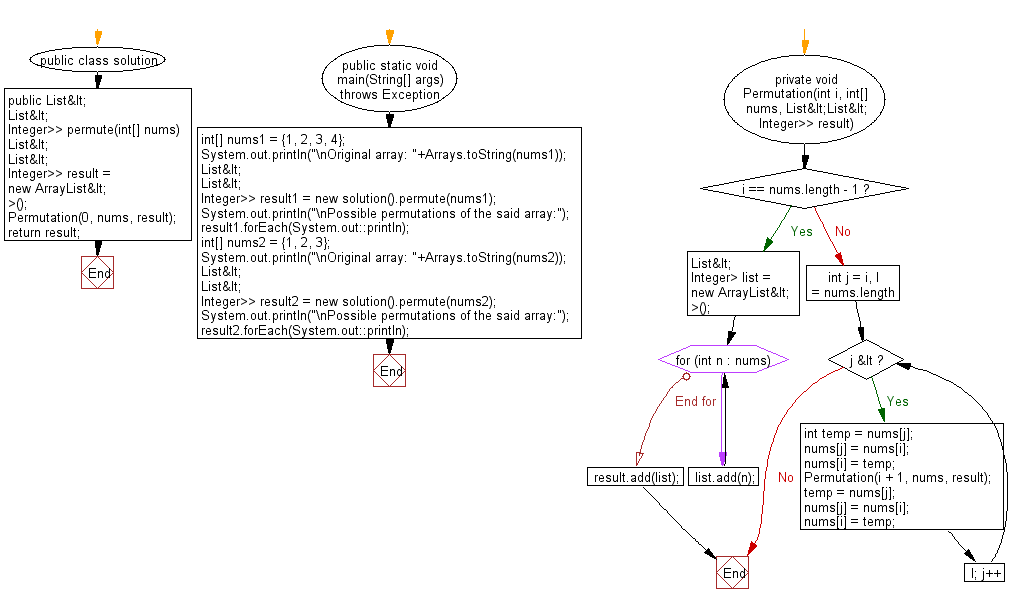﻿ Java: Possible permutations of an array of distinct integers# Java Array Exercises: Create all possible permutations of a given array of distinct integers

## Java Array: Exercise-68 with Solution

Write a Java program to create all possible permutations of a given array of distinct integers.

Example:
Input :
nums1 = {1, 2, 3, 4}
nums2 = {1, 2, 3}
Output:
Possible permutations of the said array:
[1, 2, 3, 4]
[1, 2, 4, 3]
[1, 3, 2, 4]
[1, 3, 4, 2]
....
[4, 3, 2, 1]
[4, 3, 1, 2]
[4, 1, 3, 2]
[4, 1, 2, 3]
Possible permutations of the said array:
[1, 2, 3]
[1, 3, 2]
[2, 1, 3]
[2, 3, 1]
[3, 2, 1]
[3, 1, 2]

Sample Solution:

Java Code:

``````import java.util.*;
import java.util.List;

public class solution {
public static void main(String[] args) throws Exception {
int[] nums1 = {1, 2, 3, 4};
System.out.println("\nOriginal array: "+Arrays.toString(nums1));
List<List<Integer>> result1 = new solution().permute(nums1);
System.out.println("\nPossible permutations of the said array:");
result1.forEach(System.out::println);
int[] nums2 = {1, 2, 3};
System.out.println("\nOriginal array: "+Arrays.toString(nums2));
List<List<Integer>> result2 = new solution().permute(nums2);
System.out.println("\nPossible permutations of the said array:");
result2.forEach(System.out::println);
}

public List<List<Integer>> permute(int[] nums) {
List<List<Integer>> result = new ArrayList<>();
Permutation(0, nums, result);
return result;
}

private void Permutation(int i, int[] nums, List<List<Integer>> result) {
if (i == nums.length - 1) {
List<Integer> list = new ArrayList<>();
for (int n : nums) list.add(n);
} else {
for (int j = i, l = nums.length; j < l; j++) {
int temp = nums[j];
nums[j] = nums[i];
nums[i] = temp;
Permutation(i + 1, nums, result);
temp = nums[j];
nums[j] = nums[i];
nums[i] = temp;
}
}
}
}
```
```

Sample Output:

```Original array: [1, 2, 3, 4]

Possible permutations of the said array:
[1, 2, 3, 4]
[1, 2, 4, 3]
[1, 3, 2, 4]
[1, 3, 4, 2]
[1, 4, 3, 2]
[1, 4, 2, 3]
[2, 1, 3, 4]
[2, 1, 4, 3]
[2, 3, 1, 4]
[2, 3, 4, 1]
[2, 4, 3, 1]
[2, 4, 1, 3]
[3, 2, 1, 4]
[3, 2, 4, 1]
[3, 1, 2, 4]
[3, 1, 4, 2]
[3, 4, 1, 2]
[3, 4, 2, 1]
[4, 2, 3, 1]
[4, 2, 1, 3]
[4, 3, 2, 1]
[4, 3, 1, 2]
[4, 1, 3, 2]
[4, 1, 2, 3]

Original array: [1, 2, 3]

Possible permutations of the said array:
[1, 2, 3]
[1, 3, 2]
[2, 1, 3]
[2, 3, 1]
[3, 2, 1]
[3, 1, 2]
```

Flowchart:Java Code Editor:

Improve this sample solution and post your code through Disqus

What is the difficulty level of this exercise?

Test your Programming skills with w3resource's quiz.

﻿

## Java: Tips of the Day

System.currentTimeMillis vs System.nanoTime

In Java, there are two standard ways of conducting time-operations and it is not always clear which one should be chosen.

The method System.currentTimeMillis() returns the current number of milliseconds since the beginning of the Unix era in the format Long. Its accuracy ranges from 1 to 15 thousandths of a second, depending on the system.

`long startTime = System.currentTimeMillis();long estimatedTime = System.currentTimeMillis() - startTime;`

The method System.nanoTime has an accuracy of up to one-millionth of a second (nanoseconds) and returns the current value of the most accurate available system timer.

```long startTime = System.nanoTime();long estimatedTime = System.nanoTime() - startTime;
```

Thus, it is System.currentTimeMillisexcellent for displaying and synchronizing absolute time, and System.nanoTime for measuring relative intervals.

Ref: https://bit.ly/2W4V97w